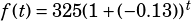Can you do Test 2 Section 3 #14? Thanks!

The thing to know here is that the basic form for exponential growth or decay is this:When this question tells you that the substance decays at an annual rate of 13%, that means you have a growth rate of –0.13. The question also tells you that the starting amount is 325 grams and that the period in years is represented by.

We can plug the given information into the standard form: